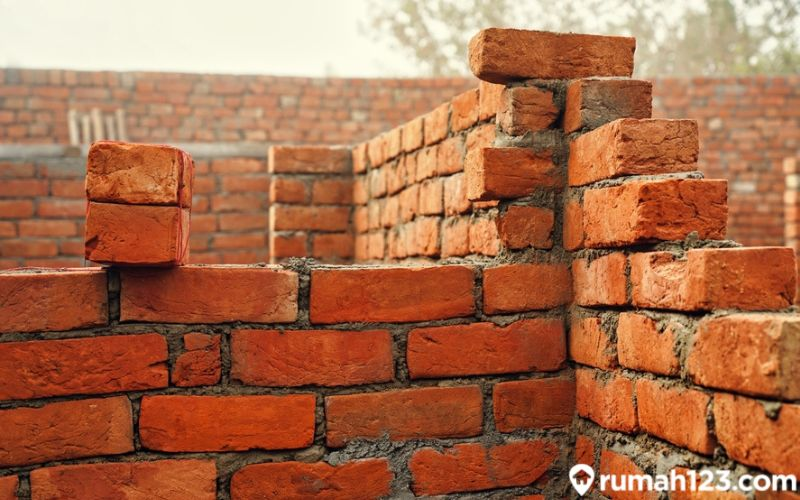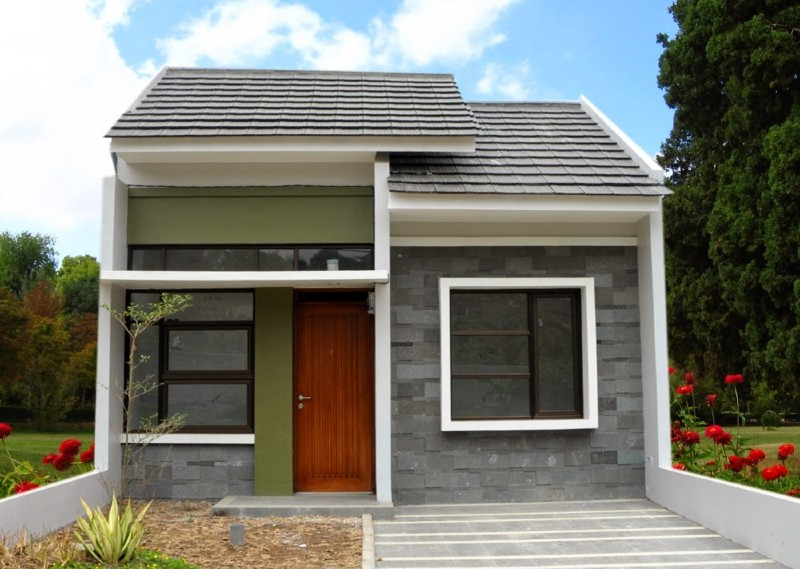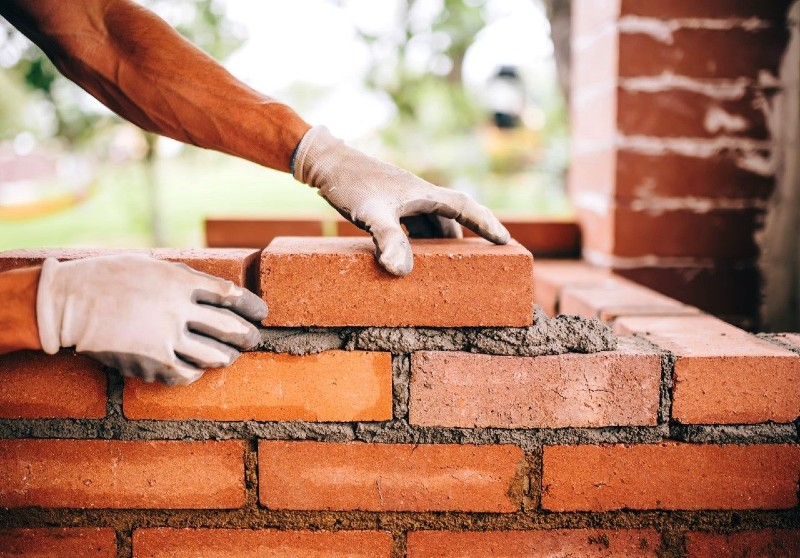# How to calculate the need for Red Brick per square meter. Completed!

How to calculate the need for Red Brick per square meter. Completed!In order to match what is needed, Property People need to know how to calculate the need for red bricks. Here’s the discussion!

Red brick is currently a widely used building material. In addition to having a strong nature, the red brick can also give a natural impression to the house.

Using red brick in the house, of course, should not be done without care. Why Property People need to do the right calculations.

If not, the need for red bricks could be more or less. Of course, this can be difficult in the development process, right?

Reporting from different sources, how to calculate the need for red bricks is easy to do, Property People just need to follow the formula below!

## How to calculate the need of Red Brick for a type 36. HouseSource: Komunitas.sikatabis.com

### 1. Calculate the perimeter of the walls of the building

For a house of type 36, the first step is to calculate the perimeter of the wall of the building. Let’s take an example for a house with a size of 6 x 6 m. Here is the formula:

Circumference (K) = 2p + 2l

K = 2(6) + 2(6)

K = 24 m.

### 2. Wall area

To get a clearer picture, also calculate the area of ​​the wall.

Area (L) = K x H (height) of the house

L = 24 m x 4 m = 96 m2.

### 3. Calculating Red Brick Needs

The last step is to calculate the number of red bricks needed. For example, if Property People uses red bricks brand AJ, in 1 square meter it takes about 60 bricks.

The calculation will be as follows:

96 m2 x 60 pieces/m2 = 5,760 pieces.

For the record, this amount is for the exterior walls only, not including the bulkhead and interior walls.

What about the cost? Property People only need to calculate the wall space with the price per piece.

## How to calculate the need of Red Brick for a house of 45 types

### 1. Calculate the perimeter of the walls of the building

Here is how to calculate the need for red bricks for the construction of walls with an area of ​​6 x 9 m.

Circumference (K) = 2p + 2l

K = 2(6) + 2(9)

K = 30 m.

### 2. Wall area

To calculate the area of ​​the wall, the People simply multiply the circumference and the height of the wall. If the height of the wall is 5 meters, the calculation is:

Area (L) = K x H house

L = 30 m x 5 m

L = 150 m2.

Thus, the number of red bricks needed for the house is 150 m2 x 60 bricks / m2 = 9000 red bricks.

## How to Calculate the Need for Red Brick for a House FenceSource: Hariankami.com

The calculation of the need for red bricks for the walls of the house and the fences is of course different. In order not to be confused, see the formula and the example of the calculation below, OK!

See also  12 ways to install an exhaust fan in the room and steps!

### 1. Measure the Fence

The first step is to find out the length and width of the fence first. For example, you have a fence that is 3 meters long, 30 cm wide and 1 meter high.

After that, you can calculate the volume of the fence using the formula:

Length x width x height

= 12 x 0.3 x 1 m

= 3.6 cubic meters.

### 2. Volume of Spices Brick and Red Mortar

The spices are a mixture of cement and sand materials that are usually used as adhesives.

To find out the need for red brick for the fence, we recommend adding a distance of about 1.2 cm for the type of mortar.

For example, buy a red brick with a size of 21 cm x 10 cm x 5.5 cm, to calculate the volume, try to add about 1.2 cm, then the size of the red brick and its specifications become:

21cm x 10cm x 6.7cm.

Meanwhile, to calculate the volume (in meters), Property People can follow the example below.

Length x width x height

= 21 cm x 10 cm x 6.7 cm

= 0.21 m x 0.1 m x 0.067 m

= 0.001407 cubic meters

### 3. Calculating the Number of Red Brick Needs

If you have found the volume, you can calculate the number of bricks needed for the fence.

There is no need to be confused, because Property People only need to divide the volume of the fence by the volume of the red brick.

Volume pagar: volume bata merah

= 3.6 cubic meters: 0.001407 cubic meters

= 2558.635394 red bricks

If rounded, then the need for red bricks becomes 2560 pieces.

However, it should be noted that this requirement only applies to fences with a width of 30 cm.

***

These are different ways to calculate the need for red bricks.